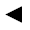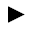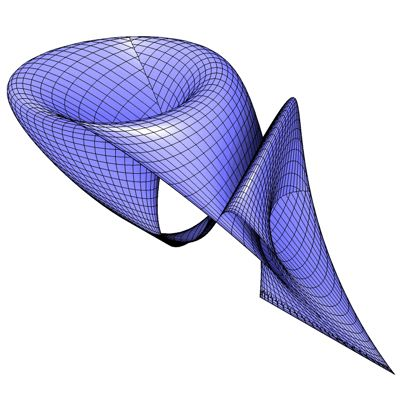[back]

## 3.49. Stiletto Surface

[next]The stiletto surface [ 8 ] is represented by the following equations.

 x = (2+cos(u)) cos(v) cos(v) cos(v) sin(v) 3-162 y = (2+cos(u+2pi/3)) cos(v+2pi/3) cos(v+2pi/3) sin(v+2pi/3) sin(v+2pi/3 ) 3-163 z = -(2+cos(u-2pi/3)) cos(v+2pi/3) cos(v+2pi/3) sin(v+2pi/3) sin(v+2pi/ 3) 3-164

To represent the area, the two parameters u and v can assume the following values ​​(domain of definition), for example.

 u is an element from the set of numbers [0, 2 pi] v is an element from the number set [0, pi]

Since the Stiletto Surface is a closed figure, the definition range must be adhered to exactly, it cannot be changed with the plugin.Fig. 53